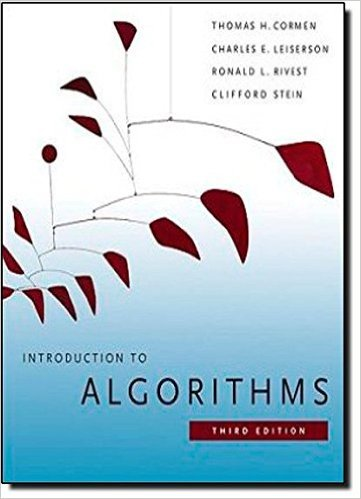×
Get Full Access to Introduction To Algorithms - 3 Edition - Chapter 23 - Problem 23-3
Get Full Access to Introduction To Algorithms - 3 Edition - Chapter 23 - Problem 23-3

×

# Bottleneck spanning tree A bottleneck spanning tree T ofISBN: 9780262033848 130

## Solution for problem 23-3 Chapter 23

Introduction to Algorithms | 3rd Edition

• Textbook Solutions
• 2901 Step-by-step solutions solved by professors and subject experts
• Get 24/7 help from StudySoup virtual teaching assistantsIntroduction to Algorithms | 3rd Edition

4 5 1 277 Reviews
23
1
Problem 23-3

Bottleneck spanning tree A bottleneck spanning tree T of an undirected graph G is a spanning tree of G whose largest edge weight is minimum over all spanning trees of G. We say that the value of the bottleneck spanning tree is the weight of the maximum-weight edge in T . a. Argue that a minimum spanning tree is a bottleneck spanning tree. Part (a) shows that finding a bottleneck spanning tree is no harder than finding a minimum spanning tree. In the remaining parts, we will show how to find a bottleneck spanning tree in linear time. b. Give a linear-time algorithm that given a graph G and an integer b, determines whether the value of the bottleneck spanning tree is at most b. c. Use your algorithm for part (b) as a subroutine in a linear-time algorithm for the bottleneck-spanning-tree problem. (Hint: You may want to use a subroutine that contracts sets of edges, as in the MST-REDUCE procedure described in 23-2.)

Step-by-Step Solution:
Step 1 of 3

LESSON 6.1b – Rational Inequalities EXAMPLE1. Solvetherationalinequality.Expressyouranswerin intervalnotation. 5x/(x–7)≥0 (Sincewehaveafractionononesideofthe equation,andazeroontheotherside,wecan setthenumeratorequaltozeroandthe denominatorequaltozero.) 5x=0 x–7=0 x=0 x=7 (Nowthatwe’vefoundthezeroes,wecan visualizetheintervalinwhichthiswouldbe

Step 2 of 3

Step 3 of 3

##### ISBN: 9780262033848

This full solution covers the following key subjects: spanning, tree, bottleneck, Linear, weight. This expansive textbook survival guide covers 35 chapters, and 151 solutions. Since the solution to 23-3 from 23 chapter was answered, more than 315 students have viewed the full step-by-step answer. Introduction to Algorithms was written by and is associated to the ISBN: 9780262033848. The full step-by-step solution to problem: 23-3 from chapter: 23 was answered by , our top Engineering and Tech solution expert on 11/10/17, 05:55PM. This textbook survival guide was created for the textbook: Introduction to Algorithms, edition: 3. The answer to “Bottleneck spanning tree A bottleneck spanning tree T of an undirected graph G is a spanning tree of G whose largest edge weight is minimum over all spanning trees of G. We say that the value of the bottleneck spanning tree is the weight of the maximum-weight edge in T . a. Argue that a minimum spanning tree is a bottleneck spanning tree. Part (a) shows that finding a bottleneck spanning tree is no harder than finding a minimum spanning tree. In the remaining parts, we will show how to find a bottleneck spanning tree in linear time. b. Give a linear-time algorithm that given a graph G and an integer b, determines whether the value of the bottleneck spanning tree is at most b. c. Use your algorithm for part (b) as a subroutine in a linear-time algorithm for the bottleneck-spanning-tree problem. (Hint: You may want to use a subroutine that contracts sets of edges, as in the MST-REDUCE procedure described in 23-2.)” is broken down into a number of easy to follow steps, and 164 words.

Unlock Textbook Solution